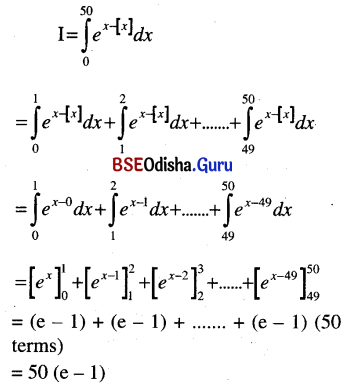# CHSE Odisha Class 12 Math Solutions Chapter 9 Integration Ex 9(k)

Odisha State Board Elements of Mathematics Class 12 Solutions CHSE Odisha Chapter 9 Integration Ex 9(k) Textbook Exercise questions and Answers.

## CHSE Odisha Class 12 Math Solutions Chapter 9 Integration Exercise 9(k)

Evaluate the following Integrals:
Question 1.
(i) $$\int_0^{\frac{\pi}{2}} \frac{d x}{1+\tan x}$$dx
Solution: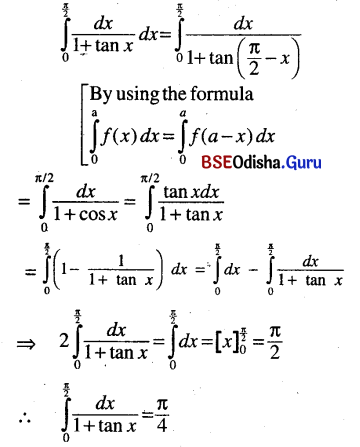(ii) $$\int_0^{\frac{\pi}{2}} \frac{\sqrt{\sin x}}{\sqrt{\sin x}+\sqrt{\cos x}}$$dx
Solution: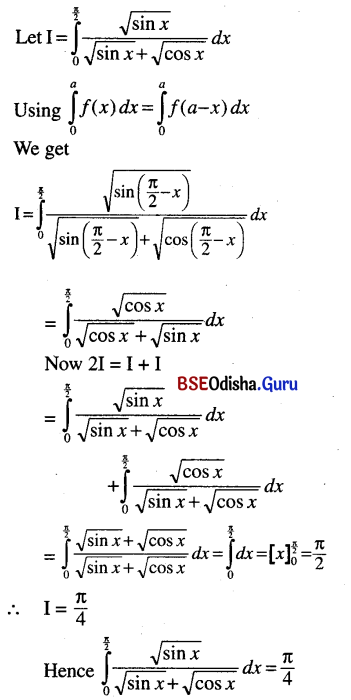(iii) $$\int_0^1 \frac{\ln (1+x)}{2+x^2}$$dx (x = tan θ)
Solution: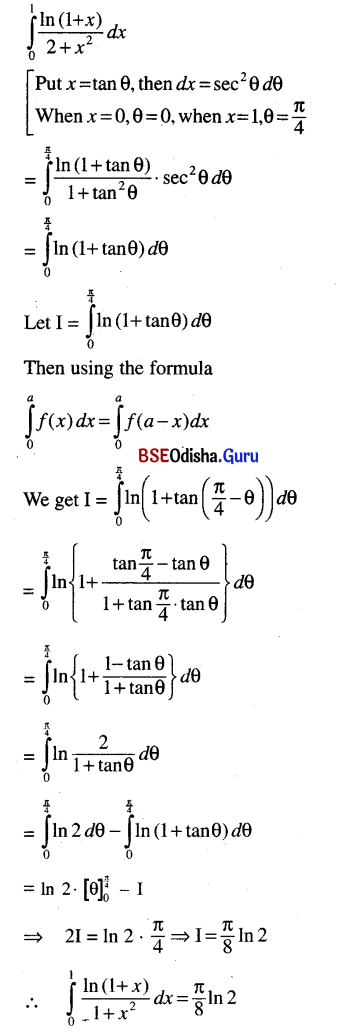(iv) $$\int_0^\pi \frac{x d x}{1+\sin x}$$
Solution: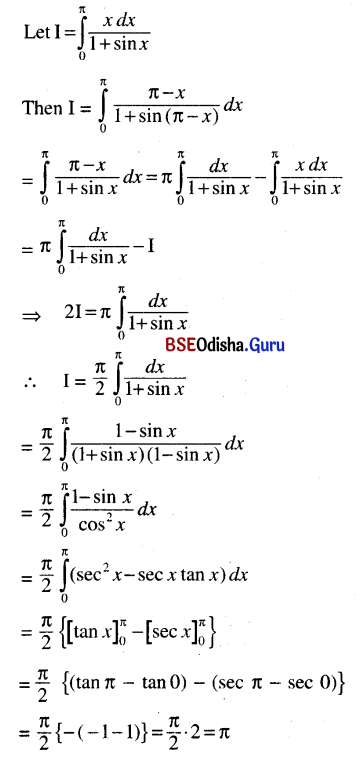Question 2.
(i) $$\int_{-a}^a$$x4 dx
Solution: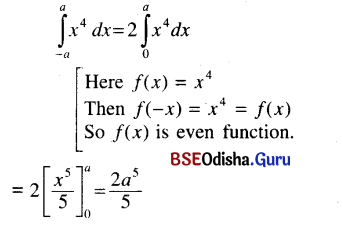(ii) $$\int_{-a}^a$$(x5 + 2x2 + x) dx
Solution: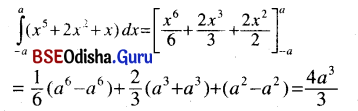(iii) $$\int_{-\frac{\pi}{4}}^{\frac{\pi}{4}}$$cos2 x dx
Solution: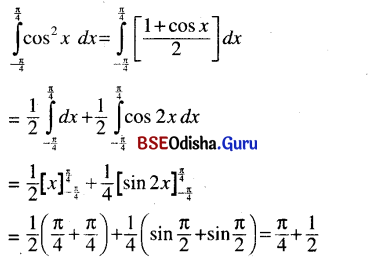(iv) $$\int_{-\frac{\pi}{6}}^{\frac{\pi}{6}}$$sin5 x dx
Solution:
Let f(x) = sin5 x
Then f(-x) = sin5 (-x)
= -sin5 x = -f(x)
So f(x) is an odd function.
Thus $$\int_{-a}^a$$f(x) dx = 0
$$\int_{-\frac{\pi}{6}}^{\frac{\pi}{6}}$$sin5 x dx = 0

Question 3.
(i) $$\int_0^\pi$$cos3 x dx
Solution:(ii) $$\int_0^\pi$$cos2 x dx
Solution: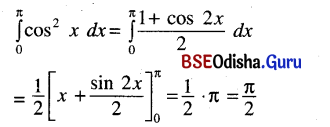(iii) $$\int_0^\pi$$sin3 x cos x dx
Solution:
$$\int_0^\pi$$sin3 x cos x dx
[Put sin x = t, then cos x dx = dt
When x = 0, t = 0, when x = π, t = 0
$$\int_0^\pi$$t3 dt = 0

(iv) $$\int_0^\pi$$sin x cos2 x dx
Solution: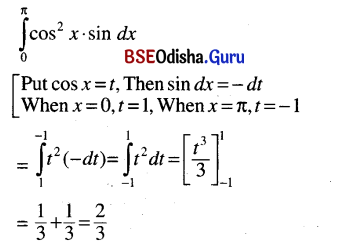Question 4.
Show that
(i) $$\int_0^1 \frac{\ln x}{\sqrt{1-x^2}}$$ dx = $$\frac{\pi}{2} \ln \frac{1}{2}$$
Solution: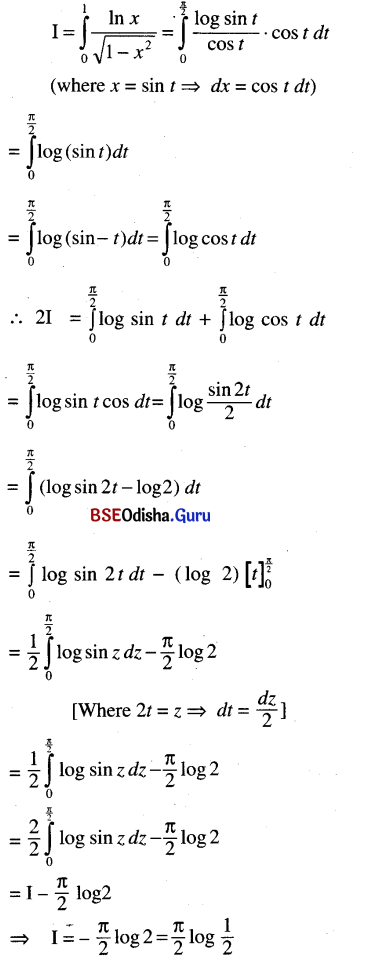(ii) $$\int_0^{\frac{\pi}{2}} \frac{\cos x-\sin x}{1+\sin x \cos x}$$ dx = 0
Solution: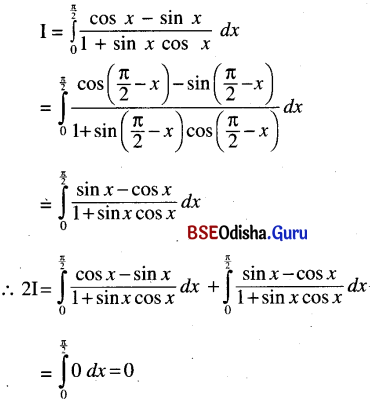(iii) $$\int_0^\pi$$x ln sin x dx = $$\frac{\pi^2}{2} \ln \frac{1}{2}$$
Solution: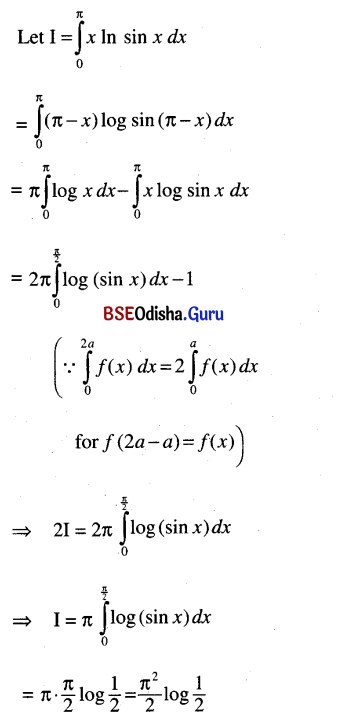Question 5.
(i) $$\int_0^{\pi / 2}$$ln (tan x + cot x) dx
Solution: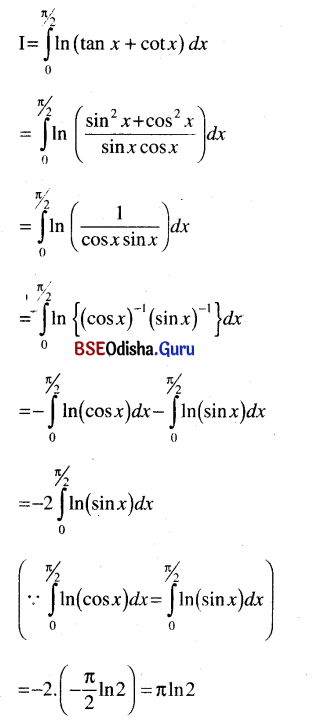(ii) $$\int_0^\pi \frac{x \tan x-\sin x}{1+\sin x \cos x}$$ dx
Solution: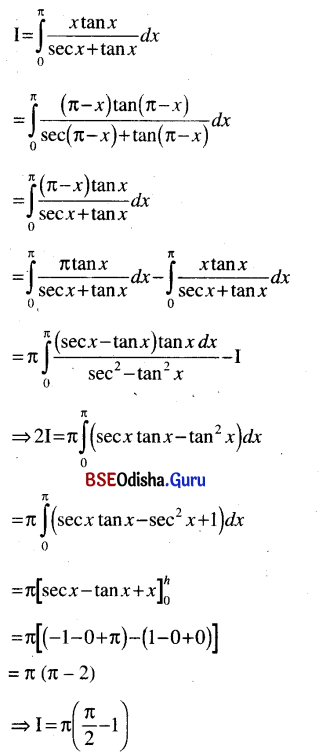(iii) $$\int_1^3 \frac{\sqrt{x} d x}{\sqrt{4-x}+\sqrt{x}}$$
Solution: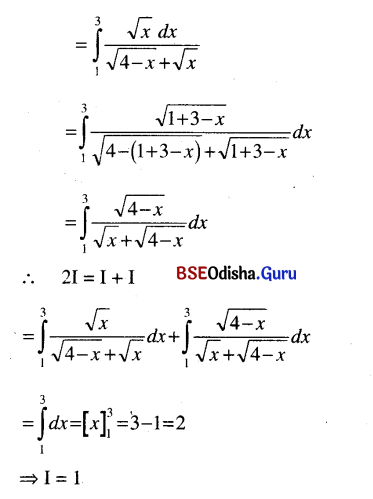(iv) $$\int_0^\pi \frac{x \sin x d x}{1+\cos ^2 x}$$
Solution: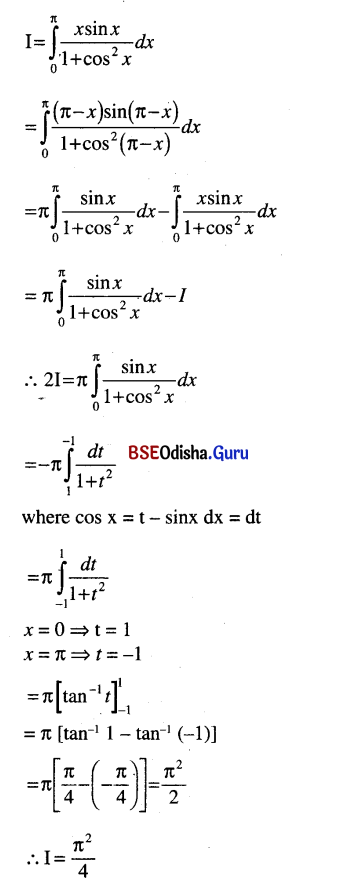(v) $$\int_0^1$$x (1 – x)100 dx
Solution: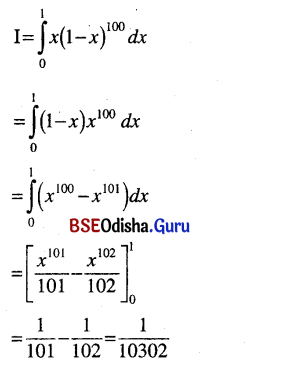(vi) $$\int_{\pi / 6}^{\pi / 3} \frac{d x}{1+\sqrt{\cot x}}$$
Solution: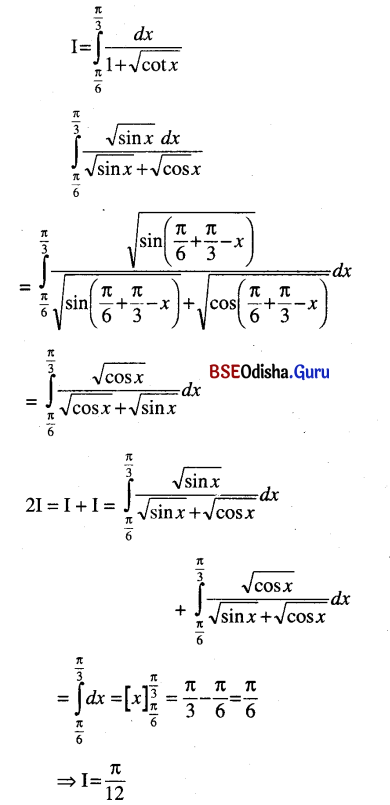(vii) $$\int_0^{50}$$ex-[x] dx
Solution: